Courses

# Facts that Matter- Circles Class 9 Notes | EduRev

## Mathematics (Maths) Class 9

Created by: Full Circle

## Class 9 : Facts that Matter- Circles Class 9 Notes | EduRev

The document Facts that Matter- Circles Class 9 Notes | EduRev is a part of the Class 9 Course Mathematics (Maths) Class 9.
All you need of Class 9 at this link: Class 9

Facts that Matter

• Equal chords of a circle (or of congruent circles) subtend equal angles at the centre.
• If two chords of a circle subtend equal angles at the centre, then the chords are equal.
• The line drawn through the centre of a circle to bisect a chord is perpendicular to the chord.
• The perpendicular from the centre of a circle to a chord bisects the chord.
• Equal chords of a circle are equidistant from the centre whereas the equidistant chords from the centre are equal.
• Chords corresponding to equal arcs are equal.
• Congruent arcs of a circle subtend equal angles at the centre.
• The angle subtended by an arc at the centre is double the angle subtended by it at any point on the remaining part.
• Angles in the same segment are equal, whereas the angle in a semicircle is a right angle.
• The sum of either pair of opposite angles of a cyclic quadrilateral is 180º.
• If the opposite angles of a quadrilateral are supplementary, then the quadrilateral is cyclic.
• If a line segment joining two points subtends equal angles at two other points lying on the same side of the line containing the line segment, then the four points are cyclic.

CIRCLES AND ITS RELATED TERMS
The locus of a point which moves in a plane in such a manner that its distance from a given fixed point is always constant, is called a circle.
The fixed point is called the centre and constant distance is called the radius of the circle. In the figure, ‘O’ is centre and OP = r is a radius. We denote it by C(O, r).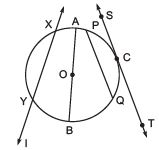A line segment, terminating (or having its end points) on the circle, is called a chord. A chord, passing through the centre is called a diameter of the circle. A line which intersects a circle in two distinct points is called a secant of the circle, whereas a line intersecting the circle in exactly one point is called a tangent to the circle.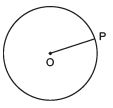In the figure,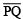is a chord,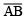is a diameter,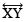is a secant and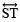is a tangent to the circle at C.

Note:
I. Diameter is the longest chord in a circle.
II. Diameter = 2 x Radius

The length of the complete circle is called its circumference, whereas a piece of a circle between two points is called an arc.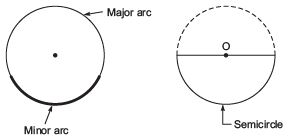Note:
I. A diameter of a circle divides it into two equal arcs, each of which is called a semicircle.
II. If the length of an arc is less than the semicircle, then it is a minor arc, otherwise, it is a major arc.

The region consisting of all points lying on the circumference of a circle and inside it is called the interior of the circle, whereas the region consisting of all points lying outside a circle is called the exterior of the circle.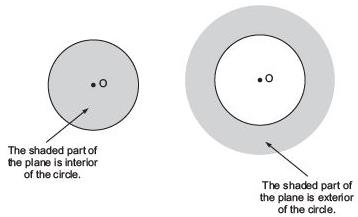Note:
The region consisting of all points which are either on the circle or lie inside the circle is called the circular region.

The part of the circular region bounded by an arc and its chord (including the chord and the arc), is called a segment of the circle. The segment containing the minor arc is called the minor segment whereas the segment containing the major arc is called the major segment.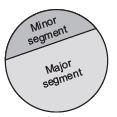,

,

,

,

,

,

,

,

,

,

,

,

,

,

,

,

,

,

,

,

,

;